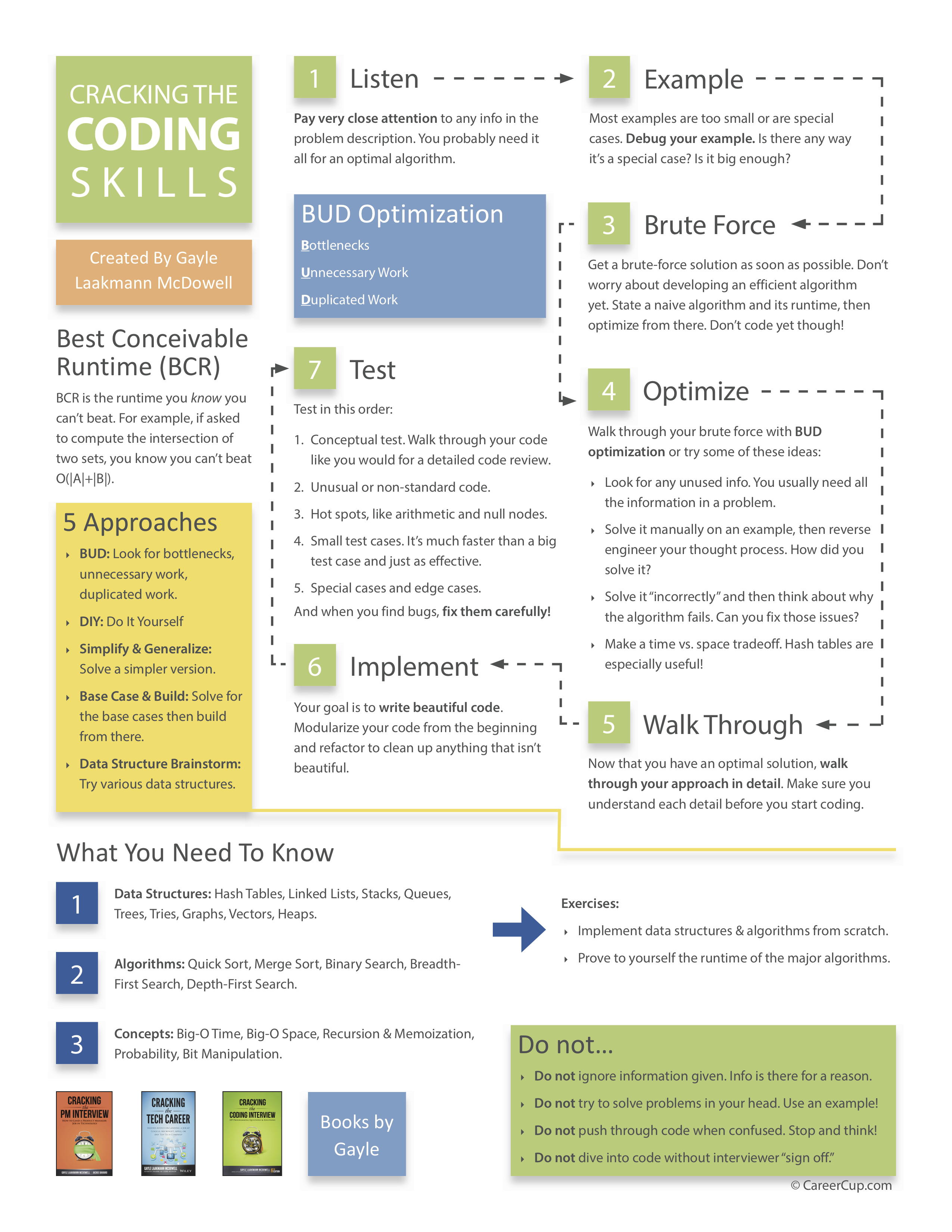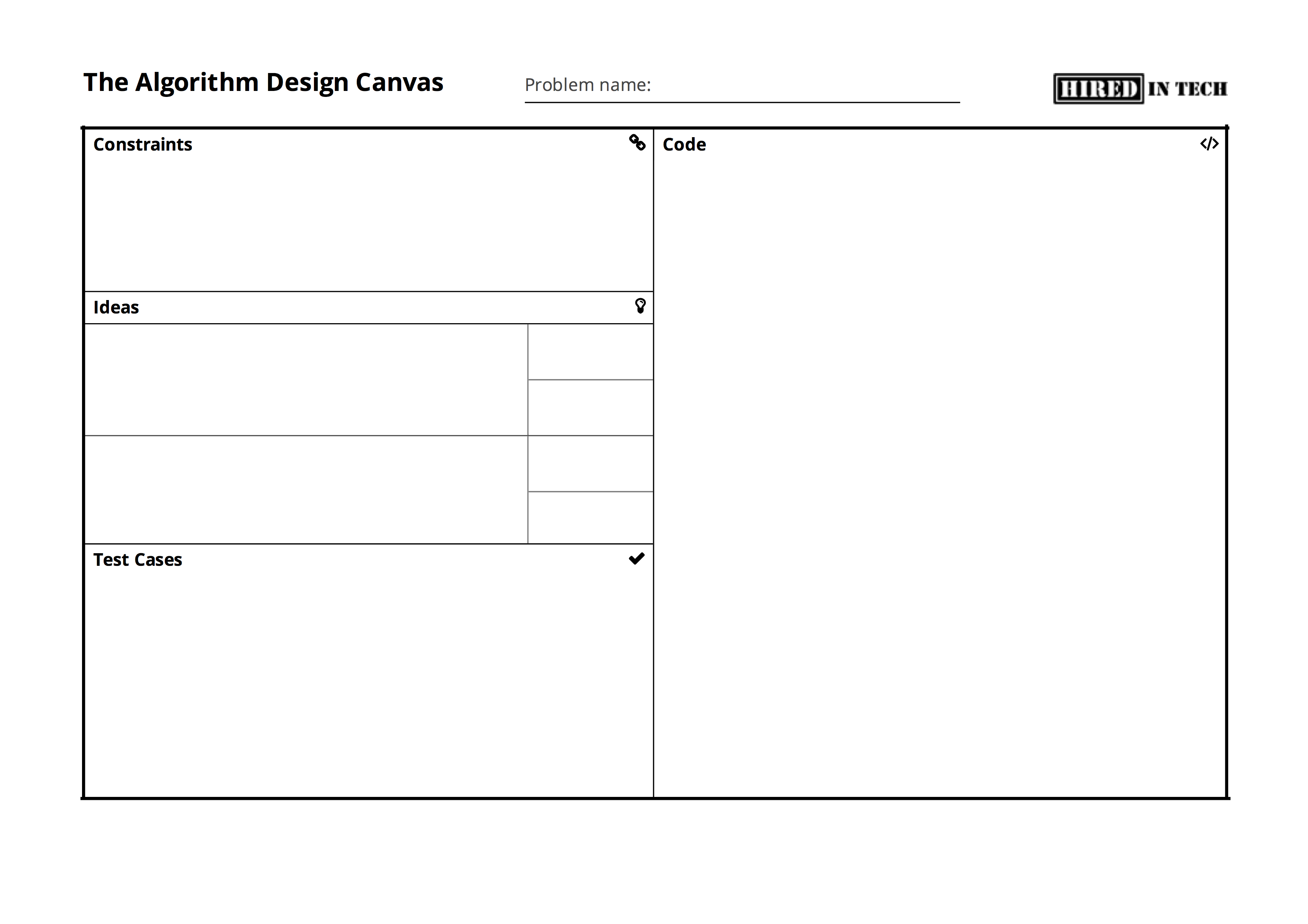Daniel Weibel
Created 1 Aug 2017
Last updated 11 Nov 2017

• Can there be floating point numbers or only integers?
• Can there be negative numbers? Zero?
• Are the numbers guaranteed to be sorted?
• Are there repeated elements?

# 2. Solve an instance of the problem manually

• The instance should be small enough to be solved manually, and big enough to see any patterns
• The instance should not be a special case
• This makes sure that you understand the question

# 3. Think about a brute force solution, if applicable

• Determine its time and space complexity

# 4. Gather ideas

• List everyting you know
• Are there any rules and patterns?
• Consider recursion
• Top-down: can the problem for $n$ be trivially solved when the solution for $n-1$ is known?
• Bottom-up: start with a base case; if you know the solution for $n=1$, can you solve the problem for $n=2$? If you know the solution for $n$, can you solve the problem for $n+1$?

# 5. Optimise the current solution

• Look for BUD (Bottlenecks, Unnecessary Work, Duplicated Work)
• Solve an instance of the problem manually and “reverse engineer” the intuitive manual approach
• Consider different data structures and algorithms
• E.g. hash table ($\text{O}(1)$ insert and delete), binary search ($\text{O}(\text{log}\,n)$), sorting ($\text{O}(n\,\text{log}\,n)$, etc.
• Goal: optimise time and space complexity by considering time vs. space tradeoffs
• Run new solution through some small test cases to confirm that it works

# 6. Implement the solution

• Write modularised code, not plain code
• That means, use method invocations instead of lengthy in-place code; you can implement the helper method later
• If necessary, use TODO comments

# 7. Test the implementation

• Run the implementation through small test cases
• Include edge cases: zero, negative numbers, empty string, empty list, etc.
• If you find a bug, fix it init in the code

# Cheatsheet

PDF versionSource: crackingthecodinginterview.com

# Algorithm Design Canvas

A generic approach for solving any algorithm design question, as proposed by HiredInTech.

PDF versionSource: hiredintech.com